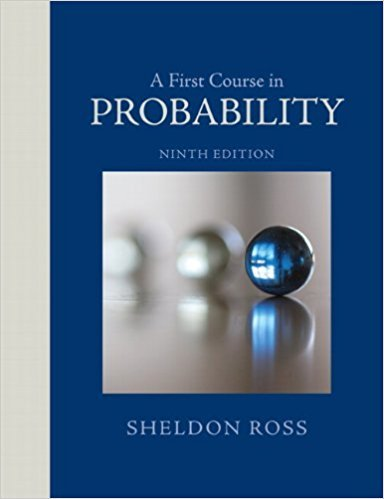×
Get Full Access to A First Course In Probability - 9 Edition - Chapter 2 - Problem 35p
Get Full Access to A First Course In Probability - 9 Edition - Chapter 2 - Problem 35p

×

# Seven balls are randomly withdrawn from an urn thatISBN: 9780321794772 63

## Solution for problem 35P Chapter 2

A First Course in Probability | 9th Edition

• Textbook Solutions
• 2901 Step-by-step solutions solved by professors and subject experts
• Get 24/7 help from StudySoup virtual teaching assistantsA First Course in Probability | 9th Edition

4 5 1 355 Reviews
19
2
Problem 35P

Problem 35P

Seven balls are randomly withdrawn from an urn that contains 12 red, 16 blue, and 18 green balls. Find the probability that

(a) 3 red, 2 blue, and 2 green balls are withdrawn;

(b) at least 2 red balls are withdrawn;

(c) all withdrawn balls are the same color;

(d) either exactly 3 red balls or exactly 3 blue balls are withdrawn.

Step-by-Step Solution:

Solution:

Step 1 of 5:

It is given that an urn contains 12 red balls, 16 blue balls and 18 green balls.

7 balls are randomly selected from the urn.

Using this we need to find the required probabilities.

Step 2 of 5

Step 3 of 5

##### ISBN: 9780321794772

This full solution covers the following key subjects: balls, withdrawn, red, Blue, Green. This expansive textbook survival guide covers 4 chapters, and 469 solutions. The answer to “Seven balls are randomly withdrawn from an urn that contains 12 red, 16 blue, and 18 green balls. Find the probability that(a) 3 red, 2 blue, and 2 green balls are withdrawn;________________(b) at least 2 red balls are withdrawn;________________(c) all withdrawn balls are the same color;________________(d) either exactly 3 red balls or exactly 3 blue balls are withdrawn.” is broken down into a number of easy to follow steps, and 58 words. A First Course in Probability was written by and is associated to the ISBN: 9780321794772. Since the solution to 35P from 2 chapter was answered, more than 1721 students have viewed the full step-by-step answer. This textbook survival guide was created for the textbook: A First Course in Probability , edition: 9. The full step-by-step solution to problem: 35P from chapter: 2 was answered by , our top Statistics solution expert on 08/11/17, 08:57AM.

Unlock Textbook Solution